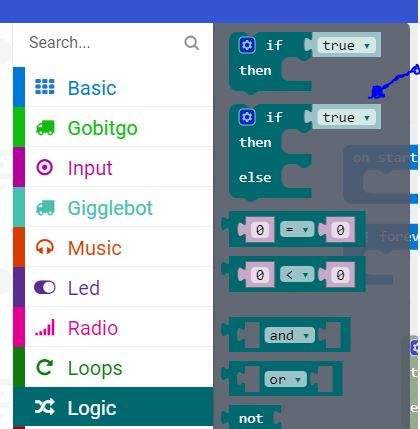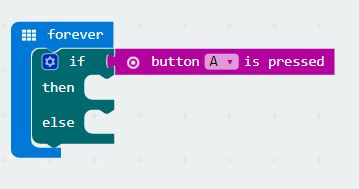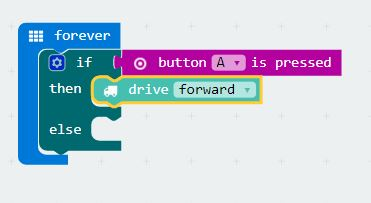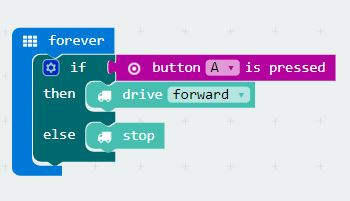GiggleBot - The Micro:Bit Robot
GiggleBot - The Micro:Bit Robot

# micro:bit Programming - Use the if then else statement in MakeCode

bbc microbit robot if then else if then else microbit robotIn this article we explore the "if then else" block in the MakeCode programming language.  This block is a logic block that extends the "if then" block.  We wrote an introduction to the "if then" block here.  In this example, we will show you how to program and if-then-else block and control a robot, helping the robot to decide if it should move forward or stop.

## Why Use the "If Then Else" block?

The if-then-else block is going to perform some logic for us:  "if something is true, then do something, if it is false, do something else".  For example:

If Button A is pressed, drive forward.  If Button A is not pressed, then Stop.
Let's see how that looks in MakeCode!

## Find the Block

The if-then-else block in makecode is located under the "Logic" area.Select it and drag it on out on the programming canvas.

## Add some Code to the If Then Else Block

Add the block, and we'll nest it under the "Forever" starting point.Let's first add some logic: is our Button A pressed?  We will add a block to find out!Now let's decide what we're going to do if the Button A is pressed, and if it is NOT pressed.

If the statement is true (the button A is pressed) we want the robot to drive forward.  That's easy enough, we'll add the GiggleBot code to drive forward.And if the statement is NOT true (the button A is NOT pressed) we want the robot to stop driving.  That's easy enough, we'll add a "stop" command under "else".Now we can download our code and put it on our robot: it should drive forward ONLY if the Button A is pressed.  And if it's not pressed, it should stop.

## Go forth!

Check out our other programming examples for the BBC micro:bit and makecode here.